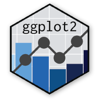Show Sidebar Hide Sidebar# geom_rug in ggplot2

How to use geom_rug with Plotly.

### New to Plotly?

Plotly's R library is free and open source!
You can set up Plotly to work in online or offline mode.
We also have a quick-reference cheatsheet (new!) to help you get started!

### Version Check

Version 4 of Plotly's R package is now available!
Check out this post for more information on breaking changes and new features available in this version.

library(plotly)
packageVersion('plotly')

##  '4.9.1'


### Basic Example

library(plotly)

df <- MASS::birthwt

df <- with(df, {
race <- factor(race, labels = c("white", "black", "other"))
ptd <- factor(ptl > 0)
ftv <- factor(ftv)
levels(ftv)[-(1:2)] <- "2+"
data.frame(low = factor(low), age, lwt, race, smoke = (smoke > 0),
ptd, ht = (ht > 0), ui = (ui > 0), ftv, bwt)
})

p <- ggplot(df, aes(lwt, bwt, colour = smoke)) +
geom_point(size = 1) +
geom_rug()

p <- ggplotly(p)

p


Reference: ggplot2 docs

### Reference